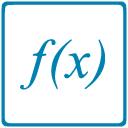Uh Oh! It seems you’re using an Ad blocker!

Since we’ve struggled a lot to makes online calculations for you, we are appealing to you to grant us by disabling the Ad blocker for this domain.# Composite Function Calculator

Enter f(x)

Enter g(x)

Enter a point:

Table of Content
 1 Domain of Composite Functions: 2 Solve the Composition of Functions (Step-by-Step): 3 What is De-Composing of a Function? 4 What is Iterated Function?
Get The Widget!

Add Composite Function Calculator to your website to get the ease of using this calculator directly. Feel hassle-free to account this widget as it is 100% free, simple to use, and you can add it on multiple online platforms.

Use an online composite function calculator that helps you to solve the composition of the functions from entered values of functions f(x) and g(x) at specific points. Also, this handy composition of functions calculator display stepwise results for composite functions f (g(x), g (f(x)), f (f(x)), and g (g(x)). Now, read on to get some extra knowledge about compositions of functions.

## What are Composite Functions?

In mathematics, composition function is an operational technique, if we have two f(x) and g(x) functions then produce a new function by composing one function into another function.

Generally, function composition is done by substitution of one function into the other function.  For Instance, g (f(x)) is the composition functions of f (x) and g (x). The composite functions g (f(x)) is pronounced as “f of g of x” or “f compose g”. Where the function f (x) is used as inner function and g (x) function is called as an outer function. Moreover, we also read g (f(x)) as “the function f (x) is inner function of the outer function g(x)”.

### Domain of Composite Functions:

The domain of composite functions g (f(x)) is always dependent on the domains of the g(x) function and the domain of the f(x) function. So, the domain is a set of all values that used in a function and a certain function must work for all given values. Also, the composite function calculator determines the function from  domain of composite functions at certain point:Example:

Find the domain for $$g(x) = \sqrt{x} and g(x) = x^2$$

Solution:
The Domain of function $$g (x) = \sqrt{x}$$ is non-negative Real Number.
The Domain of funcgtion f $$(x) = x^2$$ is the Real Number.
The composed function is:

$$(f o g)(x) = f(g(x))$$
$$= (\sqrt{x})^2$$
$$f(g(x)) = x$$

## Solve the Composition of Functions (Step-by-Step):

To determine the composition of two different functions, we use a circle (o) between the functions for composition. So f o g is pronounced as f compose g, and g o f is as g compose f respectively. Apart from this, we can plug one function into itself like f o and g o g. Here are some steps that tell how to do function composition:

• First write the composition in any form like $$(go f) (x) as g (f(x)) or (g o f) (x^2) as g (f(x^2))$$.
• Put the value of x in the outer function with the inside function then just simplify the function.

Although, you can manually determine composite functions by following these steps but to make it convenient for you. The composite function calculator will do all these compositions for you by simply enter the functions.

(Example)

## How composite function calculator works?

The composition calculator obtains the composite functions by following steps:

### Input:

• Enter the values of both f(x) and g(x) functions in specified fields.
• Now, enter a point to evaluate the compositions of functions.
• Click on the calculate button.

### Output:

After putting all the values the function composition calculator displays the following results:

• Provide the solution for composite functions (f f), (gg), (fg) and (g o f) (x).
• Give step-by-step solution at the specified point.

## FAQs:

### What is De-Composing of a Function?

The process of breaking a function into the composition of other functions. For example, $$(x+1/x^2)^4$$ this function made from composition of two functions are:

$$f(x) = x + 1/x^2$$

$$g(x) = x^4$$

And we get:

$$(g o f) (x)= g (f(x)) = g(x + 1/x) = (x + 1/x^2)^4$$

### What is Iterated Function?

The function who repeat compositions of a function with itself called iterated function like $$(g ∘ g ∘ g) (x) = g (g (g (x))) = g^3(x)$$

## Conclusion:

An online free composite function calculator that use to obtain the composition of different functions and walk through the bit-by-bit process of composing two functions. No doubt, the compositions of functions by hand is such a complex and lengthy task. But this online calculator does all these calculations numerous times without any cost for students and educators.

## Reference:

From the source of Wikipedia: Composite functions, Composition monoids, Functional powers, Composition operator.

From the source of the story of mathematics: Solve Composite Functions, Alternative notations, Multivariate functions.

From the source of PurpleMath: Composing Functions at Points, the domain of a composite function.